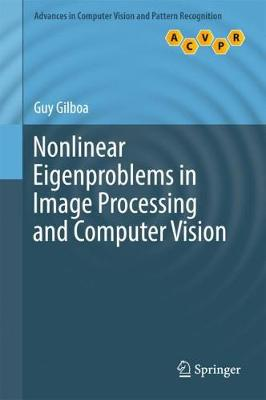•Nonlinear Eigenproblems in Image Processing and Computer Vision - Advances in Computer Vision and Pattern Recognition (Hardback)

(author)
£89.99
Hardback 172 Pages / Published: 17/04/2018
• We can order this

Usually dispatched within 3 weeks

This unique text/reference presents a fresh look at nonlinear processing through nonlinear eigenvalue analysis, highlighting how one-homogeneous convex functionals can induce nonlinear operators that can be analyzed within an eigenvalue framework. The text opens with an introduction to the mathematical background, together with a summary of classical variational algorithms for vision. This is followed by a focus on the foundations and applications of the new multi-scale representation based on non-linear eigenproblems. The book then concludes with a discussion of new numerical techniques for finding nonlinear eigenfunctions, and promising research directions beyond the convex case.

Topics and features: introduces the classical Fourier transform and its associated operator and energy, and asks how these concepts can be generalized in the nonlinear case; reviews the basic mathematical notion, briefly outlining the use of variational and flow-based methods to solve image-processing and computer vision algorithms; describes the properties of the total variation (TV) functional, and how the concept of nonlinear eigenfunctions relate to convex functionals; provides a spectral framework for one-homogeneous functionals, and applies this framework for denoising, texture processing and image fusion; proposes novel ways to solve the nonlinear eigenvalue problem using special flows that converge to eigenfunctions; examines graph-based and nonlocal methods, for which a TV eigenvalue analysis gives rise to strong segmentation, clustering and classification algorithms; presents an approach to generalizing the nonlinear spectral concept beyond the convex case, based on pixel decay analysis; discusses relations to other branches of image processing, such as wavelets and dictionary based methods.

This original work offers fascinating new insights into established signal processing techniques, integrating deep mathematical concepts from a range of different fields, which will be of great interest to all researchers involved with image processing and computer vision applications, as well as computations for more general scientific problems.

Publisher: Springer International Publishing AG
ISBN: 9783319758466
Number of pages: 172
Weight: 459 g
Dimensions: 235 x 155 mm
Edition: 1st ed. 2018